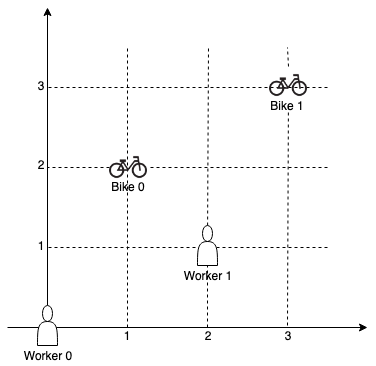##### Welcome to Subscribe On Youtube

Formatted question description: https://leetcode.ca/all/1066.html

# 1066. Campus Bikes II

Medium

## Description

On a campus represented as a 2D grid, there are N workers and M bikes, with N <= M. Each worker and bike is a 2D coordinate on this grid.

We assign one unique bike to each worker so that the sum of the Manhattan distances between each worker and their assigned bike is minimized.

The Manhattan distance between two points p1 and p2 is Manhattan(p1, p2) = |p1.x - p2.x| + |p1.y - p2.y|.

Return the minimum possible sum of Manhattan distances between each worker and their assigned bike.

Example 1:Input: workers = [[0,0],[2,1]], bikes = [[1,2],[3,3]]

Output: 6

Explanation:

We assign bike 0 to worker 0, bike 1 to worker 1. The Manhattan distance of both assignments is 3, so the output is 6.

Example 2:Input: workers = [[0,0],[1,1],[2,0]], bikes = [[1,0],[2,2],[2,1]]

Output: 4

Explanation:

We first assign bike 0 to worker 0, then assign bike 1 to worker 1 or worker 2, bike 2 to worker 2 or worker 1. Both assignments lead to sum of the Manhattan distances as 4.

Note:

1. 0 <= workers[i], workers[i], bikes[i], bikes[i] < 1000
2. All worker and bike locations are distinct.
3. 1 <= workers.length <= bikes.length <= 10

## Solution

Since the number of workers and the number of bikes are limited, this problem can be solved using dynamic programming with compressed states. For each (worker, bike) pair, calculate the Manhattan distance between the worker and the bike. Create a 2D array dp with 1 << N rows and 1 << M columns, and initialize all elements to Integer.MAX_VALUE. Then for 0 <= i < N and 0 <= j < M, set dp[1 << i][1 << j] to the distance between workers[i] and bikes[j]. Here dp[i][j] means the minimum possible sum of Manhattan distances between workers and bikes where workers in bits of i are selected and workers in bits of j are selected.

For each element dp[i][j], obtain its value using each possible worker and each possible bike, and the value is obtained from the previous state. Maintain the minimum value.

Finally, return the minimum value in the last row of dp.

• class Solution {
public int[] assignBikes(int[][] workers, int[][] bikes) {
int workersLength = workers.length, bikesLength = bikes.length;
int[][] distances = new int[workersLength][bikesLength];
for (int i = 0; i < workersLength; i++) {
int[] worker = workers[i];
for (int j = 0; j < bikesLength; j++) {
int[] bike = bikes[j];
distances[i][j] = manhattanDistance(worker, bike);
}
}
int[] ret = new int[workersLength];
boolean[] workersAssigned = new boolean[workersLength];
boolean[] bikesAssigned = new boolean[bikesLength];
for (int i = 0; i < workersLength; i++) {
int minDistance = Integer.MAX_VALUE;
int minWorkerIndex = -1, minBikeIndex = -1;
for (int j = 0; j < workersLength; j++) {
if (workersAssigned[j])
continue;
for (int k = 0; k < bikesLength; k++) {
if (bikesAssigned[k])
continue;
int distance = distances[j][k];
if (distance < minDistance) {
minDistance = distance;
minWorkerIndex = j;
minBikeIndex = k;
}
}
}
ret[minWorkerIndex] = minBikeIndex;
workersAssigned[minWorkerIndex] = true;
bikesAssigned[minBikeIndex] = true;
}
return ret;
}

public int manhattanDistance(int[] point1, int[] point2) {
return Math.abs(point1 - point2) + Math.abs(point1 - point2);
}
}

• class Solution {
public:
int assignBikes(vector<vector<int>>& workers, vector<vector<int>>& bikes) {
int n = workers.size(), m = bikes.size();
int f[n + 1][1 << m];
memset(f, 0x3f, sizeof(f));
f = 0;
for (int i = 1; i <= n; ++i) {
for (int j = 0; j < 1 << m; ++j) {
for (int k = 0; k < m; ++k) {
if (j >> k & 1) {
int d = abs(workers[i - 1] - bikes[k]) + abs(workers[i - 1] - bikes[k]);
f[i][j] = min(f[i][j], f[i - 1][j ^ (1 << k)] + d);
}
}
}
}
return *min_element(f[n], f[n] + (1 << m));
}
};

• class Solution:
def assignBikes(self, workers: List[List[int]], bikes: List[List[int]]) -> int:
n, m = len(workers), len(bikes)
f = [[inf] * (1 << m) for _ in range(n + 1)]
f = 0
for i, (x1, y1) in enumerate(workers, 1):
for j in range(1 << m):
for k, (x2, y2) in enumerate(bikes):
if j >> k & 1:
f[i][j] = min(
f[i][j],
f[i - 1][j ^ (1 << k)] + abs(x1 - x2) + abs(y1 - y2),
)
return min(f[n])


• func assignBikes(workers [][]int, bikes [][]int) int {
n, m := len(workers), len(bikes)
f := make([][]int, n+1)
const inf = 1 << 30
for i := range f {
f[i] = make([]int, 1<<m)
for j := range f[i] {
f[i][j] = inf
}
}
f = 0
for i := 1; i <= n; i++ {
for j := 0; j < 1<<m; j++ {
for k := 0; k < m; k++ {
if j>>k&1 == 1 {
d := abs(workers[i-1]-bikes[k]) + abs(workers[i-1]-bikes[k])
f[i][j] = min(f[i][j], f[i-1][j^(1<<k)]+d)
}
}
}
}
ans := inf
for _, x := range f[n] {
ans = min(ans, x)
}
return ans
}

func min(a, b int) int {
if a < b {
return a
}
return b
}

func abs(x int) int {
if x < 0 {
return -x
}
return x
}

• function assignBikes(workers: number[][], bikes: number[][]): number {
const n = workers.length;
const m = bikes.length;
const inf = 1 << 30;
const f: number[][] = new Array(n + 1)
.fill(0)
.map(() => new Array(1 << m).fill(inf));
f = 0;
for (let i = 1; i <= n; ++i) {
for (let j = 0; j < 1 << m; ++j) {
for (let k = 0; k < m; ++k) {
if (((j >> k) & 1) === 1) {
const d =
Math.abs(workers[i - 1] - bikes[k]) +
Math.abs(workers[i - 1] - bikes[k]);
f[i][j] = Math.min(f[i][j], f[i - 1][j ^ (1 << k)] + d);
}
}
}
}
return Math.min(...f[n]);
}